# Apriori Algorithm# Apriori Algorithm

Apriori algorithm is given by R. Agrawal and R. Srikant in 1994 for finding frequent itemsets in a dataset for boolean association rule. Name of the algorithm is Apriori because it uses prior knowledge of frequent itemset properties. We apply an iterative approach or level-wise search where k-frequent itemsets are used to find k+1 itemsets.

To improve the efficiency of level-wise generation of frequent itemsets, an important property is used called Apriori property which helps by reducing the search space.

Apriori Property –
All non-empty subset of frequent itemset must be frequent. The key concept of Apriori algorithm is its anti-monotonicity of support measure. Apriori assumes that

All subsets of a frequent itemset must be frequent(Apriori propertry).
If an itemset is infrequent, all its supersets will be infrequent.

Before we start understanding the algorithm, go through some definitions which are explained in my previous post.
Consider the following dataset and we will find frequent itemsets and generate association rules for them.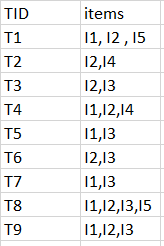minimum support count is 2
minimum confidence is 60%

Step-1: K=1
(I) Create a table containing support count of each item present in dataset – Called C1(candidate set)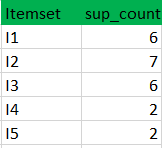(II) compare candidate set item’s support count with minimum support count(here min_support=2 if support_count of candidate set items is less than min_support then remove those items). This gives us itemset L1.Step-2: K=2

• Generate candidate set C2 using L1 (this is called join step). Condition of joining Lk-1 and Lk-1 is that it should have (K-2) elements in common.
• Check all subsets of an itemset are frequent or not and if not frequent remove that itemset.(Example subset of{I1, I2} are {I1}, {I2} they are frequent.Check for each itemset)
• Now find support count of these itemsets by searching in dataset.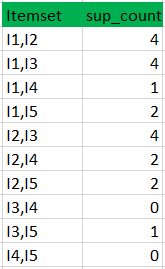(II) compare candidate (C2) support count with minimum support count(here min_support=2 if support_count of candidate set item is less than min_support then remove those items) this gives us itemset L2.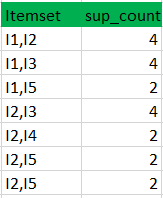Step-3:

• Generate candidate set C3 using L2 (join step). Condition of joining Lk-1 and Lk-1 is that it should have (K-2) elements in common. So here, for L2, first element should match.
So itemset generated by joining L2 is {I1, I2, I3}{I1, I2, I5}{I1, I3, i5}{I2, I3, I4}{I2, I4, I5}{I2, I3, I5}
• Check if all subsets of these itemsets are frequent or not and if not, then remove that itemset.(Here subset of {I1, I2, I3} are {I1, I2},{I2, I3},{I1, I3} which are frequent. For {I2, I3, I4}, subset {I3, I4} is not frequent so remove it. Similarly check for every itemset)
• find support count of these remaining itemset by searching in dataset.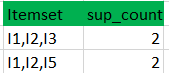(II) Compare candidate (C3) support count with minimum support count(here min_support=2 if support_count of candidate set item is less than min_support then remove those items) this gives us itemset L3.Step-4:

• Generate candidate set C4 using L3 (join step). Condition of joining Lk-1 and Lk-1 (K=4) is that, they should have (K-2) elements in common. So here, for L3, first 2 elements (items) should match.
• Check all subsets of these itemsets are frequent or not (Here itemset formed by joining L3 is {I1, I2, I3, I5} so its subset contains {I1, I3, I5}, which is not frequent). So no itemset in C4
• We stop here because no frequent itemsets are found further

• Thus, we have discovered all the frequent item-sets. Now generation of strong association rule comes into picture. For that we need to calculate confidence of each rule.

Confidence –
A confidence of 60% means that 60% of the customers, who purchased milk and bread also bought butter.

`Confidence(A->B)=Support_count(A∪B)/Support_count(A)`

So here, by taking an example of any frequent itemset, we will show the rule generation.
Itemset {I1, I2, I3} //from L3
SO rules can be
[I1^I2]=>[I3] //confidence = sup(I1^I2^I3)/sup(I1^I2) = 2/4*100=50%
[I1^I3]=>[I2] //confidence = sup(I1^I2^I3)/sup(I1^I3) = 2/4*100=50%
[I2^I3]=>[I1] //confidence = sup(I1^I2^I3)/sup(I2^I3) = 2/4*100=50%
[I1]=>[I2^I3] //confidence = sup(I1^I2^I3)/sup(I1) = 2/6*100=33%
[I2]=>[I1^I3] //confidence = sup(I1^I2^I3)/sup(I2) = 2/7*100=28%
[I3]=>[I1^I2] //confidence = sup(I1^I2^I3)/sup(I3) = 2/6*100=33%

So if minimum confidence is 50%, then first 3 rules can be considered as strong association rules.

Limitations of Apriori Algorithm

Apriori Algorithm can be moderate. The fundamental restriction is time needed to hold countless competitor sets with much regular itemsets, low least help or enormous itemsets for example it’s anything but a proficient methodology for enormous number of datasets. For instance, if there are 10^4 from regular 1-itemsets, it need to produce more than 10^7 applicants into 2-length which thus they will be tried and gather. Besides, to distinguish regular example in size 100 for example v1, v2… v100, it need to produce 2^100 up-and-comer itemsets that yield on exorbitant and burning through of season of competitor age. Thus, it will check for some sets from competitor itemsets, additionally it will filter information base ordinarily over and over for discovering up-and-comer itemsets. Apriori will be exceptionally low and shortcoming when memory limit is restricted with enormous number of exchanges.